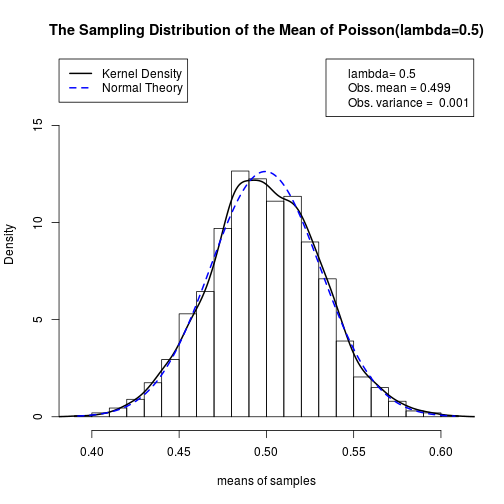``````## poisson-4.R
## Date: 2013-02-11
## Author: Paul E. Johnson <pauljohn at ku.edu>

## See the central limit theorem as it applies to a poisson
## distribution

## Run this a few times, changing the value of ell and
## sampleSize at the top. What do you see?

## Reminder: the Expected Value of a Poisson distribution equals
## the lambda coefficient

##------------------------------------------##
## ---------RUN THIS PART FIRST -------------#

ell <- 0.5
sampleSize <- 500
nOfRuns <- 2000
set.seed(123123)

getMeans <- function (x){
e <- rpois(sampleSize, lambda = ell)
mean(e)
}

samplingDist <- sapply(1:nOfRuns, getMeans)

## If you want pictures to show your parents, change SAVEME to TRUE
## Throws all images into one file (lazy).
SAVEME <- FALSE
if (SAVEME) pdf(file = "distributions-poisson-04-1.pdf", height = 10, width = 7,
paper = "special", family = "Times")

mytitle <- paste("The Sampling Distribution of a Poisson(lambda=", ell,"), N=", sampleSize)
hist(samplingDist, breaks = 10, main = mytitle)
````````````##------------------------------------------------##
# ---------- SECOND PART STARTS HERE -------------- #

# If you did not run that first part yet, go back.
# Then run this really fun part!

# Maybe you try this for fun. I've done it. It works.
op <- par(no.readonly = TRUE)
par(mfrow = c(2,1), mar = c(2.1,1,3.1,1))

z <- rpois(sampleSize, lambda = ell)

title <- paste("one sample from Poisson(lambda=",ell,")",", N=",sampleSize,sep="")
t1 <- table(z)/sampleSize

plot(t1, type = "h", xlab = "", main = title)
points(unique(as.numeric(names(t1))), as.vector(t1))

text(x = .8*max(as.numeric(names(t1))), y = .6*max(t1),
paste("E(x)=", ell, "\nObs. mean=", round(mean(z),3),
"\nObs. variance=", round(var(z),3)),
pos=2);

drawTheSamplingDist <- function(){
mytitle <-
paste("The Sampling Distribution of the Mean of Poisson(lambda=", ell,
")", sep = "")
sh <- hist(samplingDist, breaks=20, plot = F)
hist(samplingDist, prob = TRUE, breaks = 20,
xlab = "means of samples",  main = mytitle,
ylim = c(0, 1.4 * max(sh\$density)))
text(max(samplingDist), .6*max(sh\$counts),
paste("Obs. mean =",round(mean(samplingDist), 3),
"\nObs. variance = ",round(var(samplingDist), 3),
"\n", nOfRuns, "samples",
"\nSample size =", sampleSize), pos = 2)
}

## Run that a few times. It is FUN!
drawTheSamplingDist()
````````````drawTheSamplingDist()
drawTheSamplingDist()
````````````par(op)

drawTheSamplingDist()

samplingMean <- mean(samplingDist)
samplingStdDev <- sd(samplingDist)
samplingRange <- range(samplingDist)

xseq <- seq(samplingRange, samplingRange, length.out = 200)
xseqDens <- dnorm(xseq, mean = samplingMean, sd = samplingStdDev)

lines(density(samplingDist), lty = 1, lwd = 2, col = "black")
lines(xseq, xseqDens, lty = 2, lwd = 2, col = "blue")

par(xpd=TRUE)
legend("topleft", c("Kernel Density", "Normal Theory"), lty=c(1,2), lwd = 2, col = c("black","blue"))
legend("topright", c(paste("lambda=", ell),
paste("Obs. mean =",round(mean(samplingDist),3)),
paste("Obs. variance = ",round(var(samplingDist),3))))
````````````if (SAVEME) dev.off()
``````